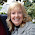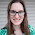Math = Love: Algebra 1 SBG Skills List 2017-2018

## Tuesday, August 8, 2017

### Algebra 1 SBG Skills List 2017-2018

Yesterday, I had the best intentions of compiling a Monday Must Reads post. But, after seven hours hanging posters in my classroom, I just wasn't feeling it. I started to beat myself up before I remembered that my goal for this year is to give myself grace.

As a result, I'm not going to try to catch up today and write a Monday Must Reads post on a Tuesday. I'll just keep collecting great blog posts and tweets for next Monday. :)

Here's a sneak peek at my favorite wall so far. Still have more posters to hang, though!

My husband blogged his newly updated SBG skill lists for Geometry and Algebra 2 today, so I thought I would do the same for Algebra 1. This will be my sixth year teaching Algebra 1. It's our second year using the Oklahoma Academic Standards which were written after Oklahoma adopted and then rejected Common Core.

After my first year of teaching to the new standards, I've figured out what worked and what utterly failed. With those things in mind, I present you my new list of Algebra 1 SBG skills:

UNIT 1 - Expressions
·         I can find the absolute value, opposite, reciprocal, and opposite reciprocal of a number.
·         I can use the order of operations to simplify expressions.
·         I can evaluate expressions. (A.3.4)
o    Include Absolute Value Bars
o    Include Fractions
·         I can evaluate expressions involving non-standard operations. (A.3.4)
·         I can rewrite expressions by combining like terms. (A.3.2)
·         I can rewrite expressions by applying the distributive property. (A.3.2)
·         I can translate between words and algebraic expressions, equations, and inequalities. (A.1.1, A.2.1)

UNIT 2 - Solving Equations and Inequalities
·         I can solve equations and inequalities with variables on one side of the equal sign. (A.1.1)
·         I can solve equations and inequalities with variables on both sides of the equal sign. (A.1.1)
·         I can solve and graph compound inequalities on a number line. (A.2.2)
·         I can solve absolute value equations. (A.1.2)
·         I can solve absolute value inequalities. (A.2.2)
·         I can solve an equation with many variables for one variable in terms of the others. (A.3.1)

UNIT 3 - Relations and Functions
·         I can generate equivalent representations of a relation and determine if the relation is a function or not. (F.1.1)
·         I can identify the independent and dependent variables in a situation. (F.1.2)
·         I can find the domain and range of a function. (F.1.2)
·         I can identify domain and range restrictions in real-life situations. (F.1.2)
·         I can read and interpret the graph of a real-life situation. (F.1.4)
o    Piecewise linear graphs – not step functions!
o    Identify Independent and Dependent Variables
o    Identify Domain and Range
o    Identify Rate of Change
·         I can create a graph to describe a story and create a story to describe a graph. (A.4.4)
·         I can evaluate functions and interpret the results in real-life contexts. (F.3.2)
·         I can write a function (using function notation) to model a real-world situation and use the function to answer questions. (F.1.3)
·         I can determine if a function is linear or non-linear (quadratic, exponential or absolute value). (F.2.1, F.2.2)
o    Emphasize Graphing by Making Input-Output Tables!

UNIT 4 - Linear Graphs and Inequalities
·         I can calculate and interpret slopes and intercepts of a linear function. (A.4.1)
·         I can classify equations as parallel, perpendicular, or neither. (A.4.2)
·         I can write an equation (in slope-intercept, point-slope, and standard form) and use the equation to solve problems. (A.4.3)
·         I can identify and generate equivalent representations of linear equations, graphs, tables, and real-world situations. (F.3.1)
·         I can predict how a linear graph will be transformed when the equation is changed. (F.2.2)
·         I can create scatterplots, determine regression and correlation, and use these to make predictions and assess the reliability of predictions. (D.1.2)
·         I can graph a linear inequality and interpret the solution. (A.2.1)
·         I can write a linear inequality and use the inequality to solve problems. (A.2.1)

UNIT 5 - Absolute Value Graphs and Inequalities
·         I can find the slope, vertex, and orientation of an absolute value relation.
·         I can write the equation or inequality for an absolute value relation.
·         I can predict how the graph of an absolute value graph or inequality will be transformed when the equation or inequality is changed. (F.2.2)

UNIT 6 - Systems of Equations and Inequalities
(With each skill, have students determine how many solutions the system has!)
·         I can solve systems of equations graphically and interpret the solution. (A.1.3)
·         I can solve systems of equations using the substitution method. (A.1.3)
·         I can solve systems of equations using the elimination method. (A.1.3)
·         I can solve systems of inequalities and graph and interpret the solution on a coordinate plane. (A.2.3)

UNIT 7 - Polynomials
·         I can name polynomials according to their degree and number of terms.
·         I can write polynomials in standard form.
·         I can add and subtract polynomials (including when written in function notation). (A.3.2, F.3.3)
·         I can multiply polynomials (including when written in function notation). (A.3.2, F.3.3)
·         I can factor out the GCF of a polynomial. (A.3.3)
·         I can fully factor a polynomial. (A.3.3)

UNIT 8 - Radicals
·         I can simplify radical expressions. (N.1.1)
·         I can add and subtract radical expressions. (N.1.2)
·         I can multiply radical expressions. (N.1.2)
·         I can divide radical expressions, rationalizing the denominator when necessary. (N.1.2)

UNIT 9 - Sequences
·         I can classify a sequence as arithmetic, geometric, fibonacci, or neither and find the next term in the sequence.
·         I can recognize that arithmetic sequences are linear and write a formula to find any term in the sequence. (A.3.5)
·         I can recognize that geometric sequences are exponential and write a formula to find any term in the sequence. (A.3.6)

UNIT 10 - Data Analysis
·         I can find the mean, median, mode, and range of a set of data.
·         I can determine if a set of data is discrete or continuous. (D.1.3)
·         I can create a data display for a set of data and use it to describe the data set. (D.1.1)
·         I can interpret a data display.
·         I can compare data sets using summary statistics. (D.1.1)

UNIT 11 - Probability
·         I can determine the size of a sample space and use it to calculate probabilities. (D.2.1)
o    Tree Diagrams
o    Counting Procedures (Multiplication and Addition Principles)
·         I can calculate experimental probabilities by performing experiments or simulations. (D.2.3)
·         I can find complements, intersections, and unions using Venn Diagrams. (D.2.2)
·         I can use Venn Diagrams to find the probability of an event (D.2.2).
·         I can apply probability concepts to real-world situations to make informed decisions (D.2.4).

The bold skills are not explicitly required by the standards, but I feel that they are necessary for students to master. I know for sure these won't be perfect either, but I'll wait until next summer to go back to the drawing board. I love that every year I get a chance to be a bit of a better teacher than the year before. I'm always improving, and I'm thankful for each one of you reading this post. You are helping me on this journey to be the best teacher possible.

1.1.